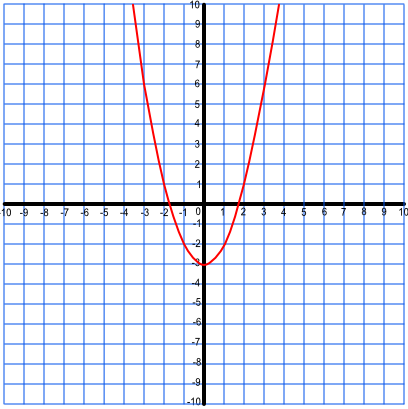GCSE(F), GCSE(H),

A quadratic function will contain a squared term, but will have no higher power. Plotted on a graph, it will be in the shape of a parabola, which is a curve that comes to a rounded point then turns to curve back again.

The point at which it turns is a turning point, and this will be either a minimum or a maximum value. Curves can fall, turn, and rise again; or they may rise, peak, and fall.

Draw graphs as accurately to obtain any turning points or roots.

## Examples

1. Plot the graph y = x2 - 3.

Answer:The graph y = x2 - 3 may be plotted using the following points:

 x -4 -3 -2 -1 0 1 2 3 4 x2 - 3 13 6 1 -2 -3 -2 1 6 13

2. For the graph, above: is the turning point a minimum value or a maximum value?